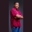Related Tags

c#
communitycreator

# How to compare two double values in C#Theodore Kelechukwu Onyejiaku

### Overview

We use the CompareTo() method to compare two double values or objects in Ruby. Remember that a double value in C# represents a double-precision, floating-point number.

When we compare two double values, say d1 and d2, the CompareTo() method returns an integer that is less than 1 if the latter is lesser than the former. It returns 0 if they are both equal, or just 1 if the latter is greater.

### Syntax

public int CompareTo (double d);
Syntax for Comparing Two Double Values

### Parameters

d: This is the decimal to compare.

### Return value

The value returned is an integer. This method returns a signed number that indicates whether a particular double instance is greater, lesser than, or equal to d. 1 is returned if the instance is greater than d. -1 is returned if the instance is lesser than d, and 0 is returned if the instance and d are equal.

### Code example

// use System
using System;
// create class
class DoubleComparer
{
// main method
static void Main()
{
// create double values
double doubleNumberOne = 23.454;
double doubleNumberTwo = doubleNumberOne * 1;
double doubleNumberThree = 45.34;

// comparing values and printing results
Console.WriteLine(doubleNumberOne.CompareTo(doubleNumberTwo));  // 0 : Equal
Console.WriteLine(doubleNumberOne.CompareTo(doubleNumberThree));  // -1 : lesser than
Console.WriteLine(doubleNumberThree.CompareTo(doubleNumberOne));  // 1 : greater than
}
}
Comparing double values in C#

### Code explanation

• Lines 10–12: We instantiate the double variables d1, d2, and d3 and initialize them with values.
• Line 15: We compare d1 to d2, and it returns 0.
• Line 16: We compare d1 to d3, and it returns -1.
• Line 17: We compare d3 to d1, and it returns 1.

RELATED TAGS

c#
communitycreator

CONTRIBUTORTheodore Kelechukwu Onyejiaku
RELATED COURSES

View all Courses

Keep Exploring

Learn in-demand tech skills in half the time